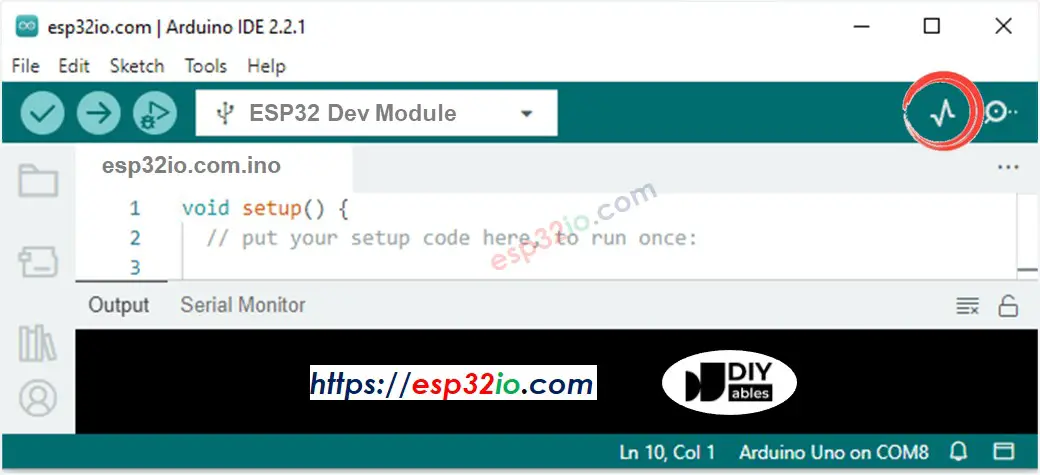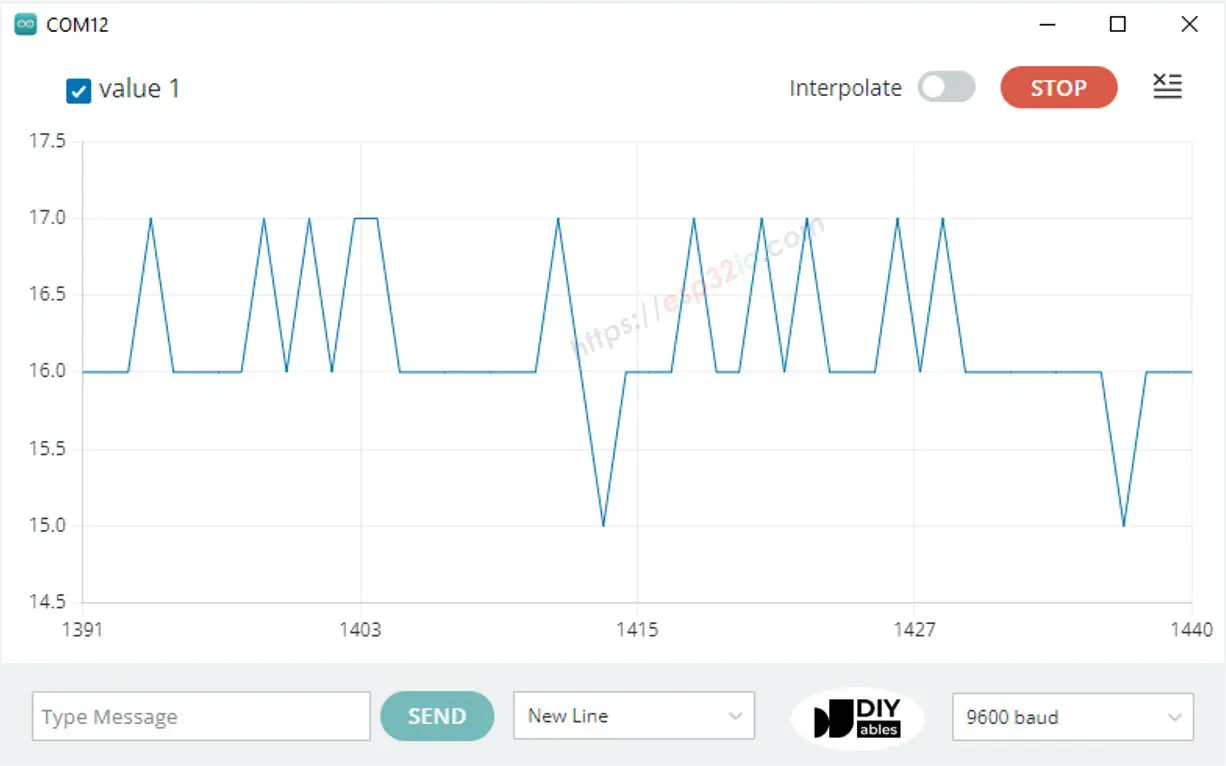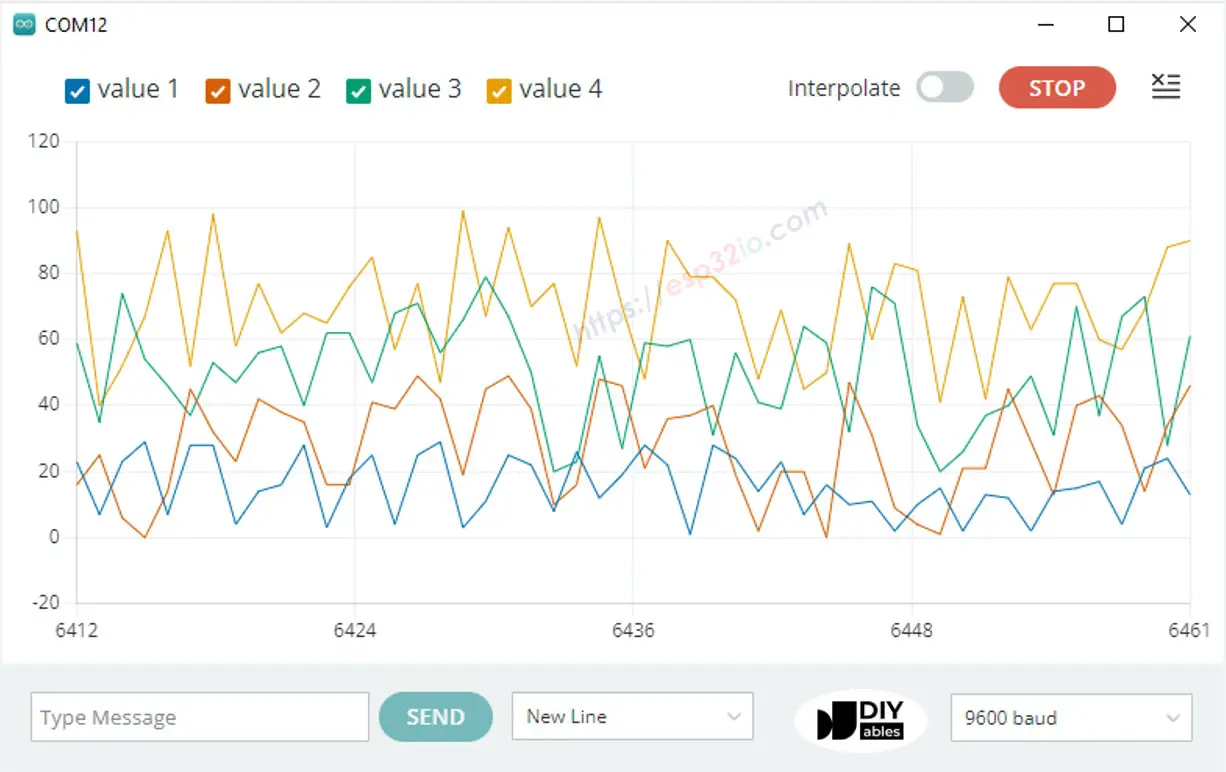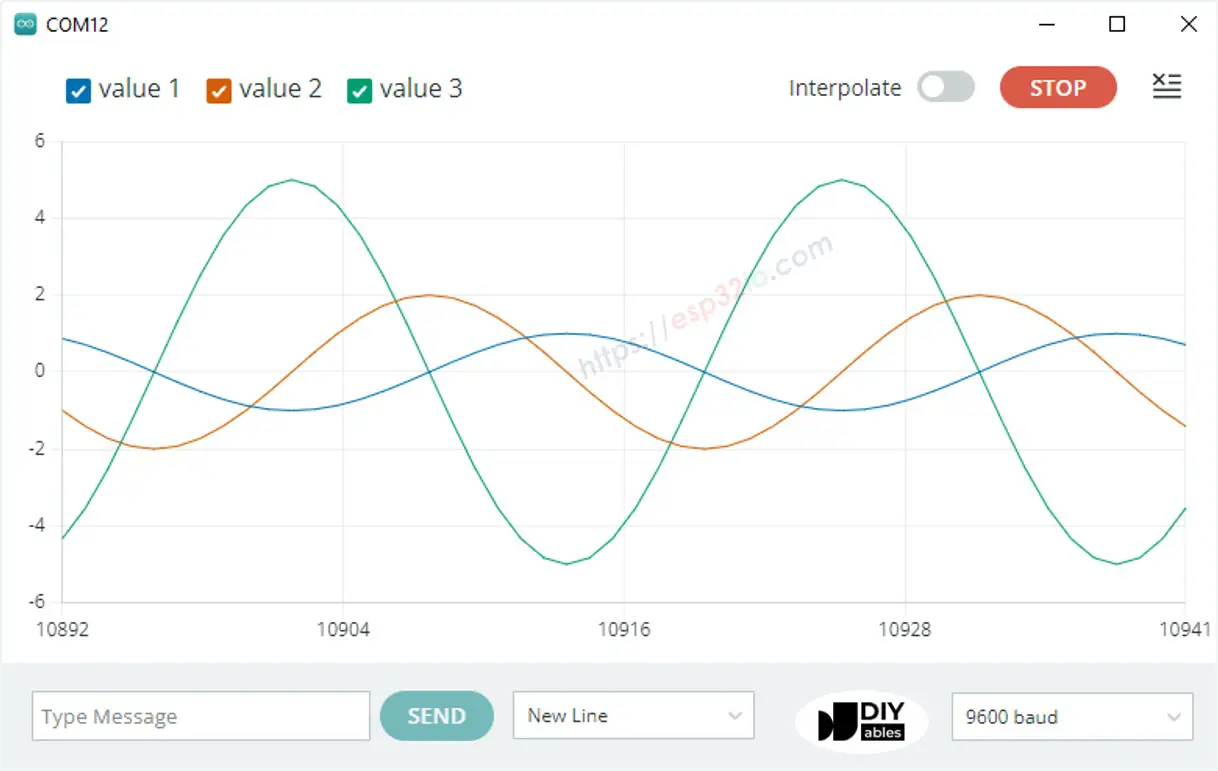# ESP32 - Serial Plotter

This tutorial instructs you how to use Serial Plotter on Arduino IDE with ESP32

## Hardware Used In This Tutorial

Disclosure: some of these links are affiliate links. We may earn a commission on your purchase at no extra cost to you. We appreciate it.

## Introduction to Serial Plotter

The Serial Plotter is a tool on Arduino IDE the can receive data from ESP32 via Serial and plot it on a graph. The Serial Plotter can plot multiple sensor's data in the same screen.

To receive the data from ESP32, It needs to use a micro USB cable between ESP32 and PC

Serial Plotter is composed of two components:

• a selection box: used to select the serial baudrate
• a graph:: a screen that shows the visualized data
• X-axis: presents the time. It has 500 points. The time between each point is the time between two consecutive Serial.println() function calls.
• Y-axis: presents the data values received from ESP32. The Y-axis automatically scales when the data's value increases or decreases.

## How To Open Serial Plotter

On Arduino IDE, Navigate to Tools Serial Plotter## How to plot a Single Line on Graph

To plot a single line on graph, we just need to send the data terminated by “\r\n” character. We can use Serial.println() function

Serial.println(variable);

※ NOTE THAT:

Serial.println() automatically appends “\r\n” characters to data.

### Example Code

The below ESP32 example code reads the value of an analog input pin and send it to Serial Plotter

/* * This ESP32 code is created by esp32io.com * * This ESP32 code is released in the public domain * * For more detail (instruction and wiring diagram), visit https://esp32io.com/tutorials/esp32-serial-plotter */ void setup() { Serial.begin(9600); } void loop() { int variable_1 = analogRead(A0); Serial.println(variable_1); delay(100); }

#### Quick Instructions

• If this is the first time you use ESP32, see how to setup environment for ESP32 on Arduino IDE.
• Copy the above code and paste it to Arduino IDE.
• Compile and upload code to ESP32 board by clicking Upload button on Arduino IDE
• Open Serial Plotter
• Select baurate 9600
• See graph on Serial Plotter## How to plot multiple lines on Graph

To plot multiple variables, we need to separate variables from each other by “\t” or " " character. The last value MUST be terminated by “\r\n” characters.

In detail:

• The first variable
Serial.print(variable_first);
• The middle variables
Serial.print("\t"); // or Serial.print(" ") Serial.print(variable_nth);
• The last variable
Serial.print("\t"); // or Serial.print(" ") Serial.println(variable_last);

### Example Code

The below ESP32 example code reads the value from 4 analog input pins and sends them to Serial Plotter

/* * This ESP32 code is created by esp32io.com * * This ESP32 code is released in the public domain * * For more detail (instruction and wiring diagram), visit https://esp32io.com/tutorials/esp32-serial-plotter */ void setup() { Serial.begin(9600); } void loop() { int variable_1 = analogRead(A0); int variable_2 = analogRead(A1); int variable_3 = analogRead(A2); int variable_4 = analogRead(A3); Serial.print(variable_1); Serial.print(" "); // a tab '\t' or space ' ' character is printed between the two values. Serial.print(variable_2); Serial.print(" "); // a tab '\t' or space ' ' character is printed between the two values. Serial.print(variable_3); Serial.print(" "); // a tab '\t' or space ' ' character is printed between the two values. Serial.println(variable_4); // the last value is terminated by a carriage return and a newline characters. delay(100); }

The result on Serial Monitor:### Example of 3 Sine Waveforms

The below ESP32 example code print three sine waveform's value to Serial Plotter

/* * This ESP32 code is created by esp32io.com * * This ESP32 code is released in the public domain * * For more detail (instruction and wiring diagram), visit https://esp32io.com/tutorials/esp32-serial-plotter */ void setup() { Serial.begin(9600); } void loop() { for (int i = 0; i < 360; i += 5) { float sine_1 = 1 * sin(i * M_PI / 180); float sine_2 = 2 * sin((i + 90) * M_PI / 180); float sine_3 = 5 * sin((i + 180) * M_PI / 180); Serial.print(sine_1); Serial.print("\t"); // a tab '\t' or space ' ' character is printed between the two values. Serial.print(sine_2); Serial.print("\t"); // a tab '\t' or space ' ' character is printed between the two values. Serial.println(sine_3); // the last value is terminated by a carriage return and a newline characters. delay(100); } }

The result on Serial Plotter:## Video Tutorial

Making video is a time-consuming work. If the video tutorial is necessary for your learning, please let us know by subscribing to our YouTube channel , If the demand for video is high, we will make the video tutorial.